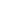Flat 50% off

Ends in

• JEE Main & Advanced
•• Mathematics
• Back to Doubt Clearing

## Let $p(n)$ be the number of digits when $8^{n}$ is written is base 6, and let $q(n)$ be the number of digits when $6^{n}$ is written in base 4. For example, $8^{2}$ in base 6 is 144, hence $p(2) = 3$. Then $lim_{n \rightarrow \infty}$ ${p(n)q(n) \over n^2}$ equals :(A) 1(B) $\frac{4}{3}$(C) $\frac{3}{2}$(D) 2

### Asked By Bhavesh KHATRI

Updated Tue, 21 May 2019 12:56 pm